# «自动机理论、语言和计算导论»笔记：第二章 有限自动机

## 2.1.1 定义

1. 一个状态集合 $Q$

2. 一个输入符号集合 $T$

3. 一个转移函数 $d$。这个函数接受一个输入字符，返回一个新的状态。

4. 一个初始状态 $q_0$

5. 一个终末状态集合 $F$

### 2.1.2 处理串

DFA 所接受的所有串的集合称作 DFA的语言

NFA 同理

### 2.1.3 DFA 的简记法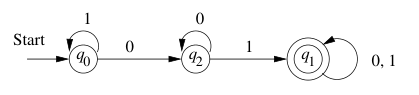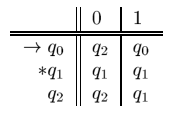$\rightarrow$ 表示初始状态。 $*$ 表示终末状态。

## 2.2 非确定有限自动机（Nondeterministic Finite Automata）

NFA 能够同时处在几个状态。因此若是识别同样的语言，NFA 能够更加简练。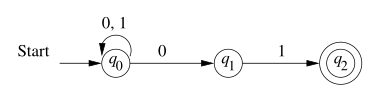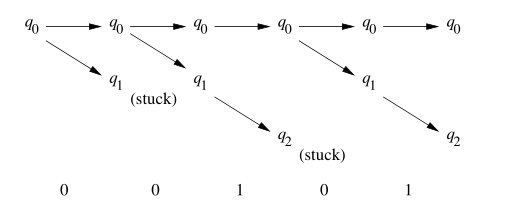### 2.2.1 定义

NFA 的定义和 DFA 的唯一区别在于，NFA 返回一个状态的集合

### 2.2.2 扩展转移函数

NFA 的扩展转移函数，返回的是状态机处理字符串 w 后处在的各状态（构成的集合）。

## 2.3 带 $\varepsilon$ 转移的 FA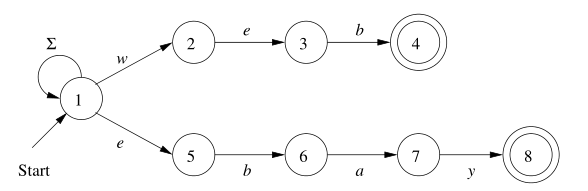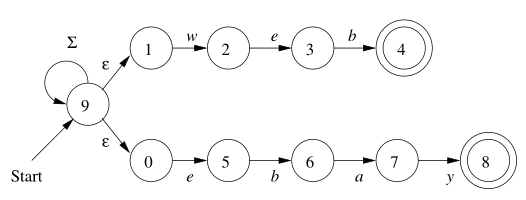### 2.3.1 $\varepsilon-\text{NFA}$ 的定义

NFA 和 $\varepsilon-\text{NFA}$ 的唯一区别在于，转移函数必须至少有一个是无条件（空输入）转移的。

important!